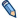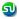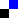accelerationacceleration defined in 1939 year

acceleration - Acceleration (Lat. accelerare, to hasten);
acceleration - Word used in its most general sense to indicate any change in velocity. Hence it may mean an increase or a decrease in the speed, or a change in the direction of motion. Commonly, however, acceleration is employed to denote a change in speed only. It may be uniform, in which case equal changes of speed occur in equal times, or it may be variable, If the speed is increasing, then the acceleration is positive: if decreasing, negative. Therefore negative acceleration is what is ordinarily called retardation. Uniform acceleration is measured by the change in speed taking place in a given time when divided by that time. The formula is thus a = (v2 - v1)/t where v2 and v1 are the respective "velocities, a the acceleration, and t the time. Variable acceleration is measured by dividing the change in velocity occurring in an interval so small that the acceleration does not appreciably change in it, by the time. See Dynamics; Motion, Laws of.

near acceleration in Knolik

 accademia della crusca homeletter "A"start from "AC" accent

definition of word "acceleration" was readed 1086 times

Legal info